# 二氧化硅与铁细菌混合污垢的趋热性分析Thermotactic Analysis of Composite Fouling Composed of SiO2 and IB

DOI: 10.12677/JAPC.2017.64019, PDF, HTML, XML, 下载: 872  浏览: 2,172

Abstract: In order to investigate the effects of temperature to composite fouling composed of SiO2 and IB, and learn about the mechanism of composite fouling in different temperatures of hot fluid loop and cool fluid loop, experiments based on thermal resistance method and weighing method were carried out in different cycle entrance temperatures and cooling entrance temperatures. It is showed that the thermal resistance of composite fouling, which changes in a regular way, is evidently affected by the entrance temperatures of hot fluid loop and cool fluid loop. Increasing the inlet temperature of the thermal cycle, the increase of the thermal resistance of the mixed fouling decreases, and the inlet temperature of the cold cycle is also positively correlated with the increment of the mixed fouling.

1. 引言

2. 实验系统及原理

2.1. 实验系统

2.2. 实验工质的制备

2.3. 实验方法

1) 安装实验台，对实验段进行杀菌处理

2) 在冷却水循环系统水箱加入清洁水，启动冷却水循环系统循环泵，进行检漏，待冷却水循环系统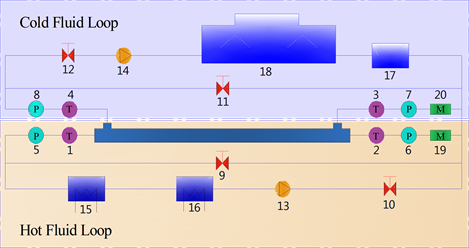1、2、3、4—温度传感器(PT100)；5、6、7、8—压力传感器；9、10、11、12—调节阀；13—热流体循环系统循环泵；14—冷却水循环系统循环泵；15—级恒温水箱；16—二级恒温水箱；17—冷却水循环水箱；18—冷水机组19、20—电磁流量计

Figure 1. Fouling resistance online monitor system in heat pump conditions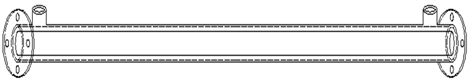Figure 2. Sleeve type heat exchanger

3) 向热流体循环系统二级恒温水箱加入400 mg/L的二氧化硅颗粒和2%的铁细菌培养液，开启PC数据采集仪进行数据采集。

4) 运行12 h后停止试验台，取出实验段的内管水平放置，并于10 h之后对内管进行称量。

5) 重新组装启动试验台，重复步骤(4)

6) 实验完毕后，对实验台进行清理，改变工况参数重复步骤(1)到(5)

2.4. 实验原理

${R}_{f1}={R}_{f}-{R}_{0}$ (1)

${Q}_{1}={Q}_{2}$ (2)

$Q=\frac{{Q}_{1}+{Q}_{2}}{2}$ (3)

${Q}_{1}={c}_{p1}{q}_{m1}\left({t}_{2}-{t}_{1}\right)$ (4)

${Q}_{2}={c}_{p2}{q}_{m2}\left({t}_{3}-{t}_{4}\right)$ (5)

$R=\frac{A\cdot \Delta T}{Q}$ (6)

$\Delta T=\frac{\left({t}_{1}-{t}_{4}\right)-\left({t}_{2}-{t}_{3}\right)}{\mathrm{ln}\frac{{t}_{1}-{t}_{4}}{{t}_{2}-{t}_{3}}}$ (7)

$\Delta {m}_{n}={m}_{n}-{m}_{0}$ (8)

3. 实验结果与分析

3.1. 热循环工质入口温度对于混合污垢的影响

3.2. 冷循环水浴入口温度对于混合污垢的影响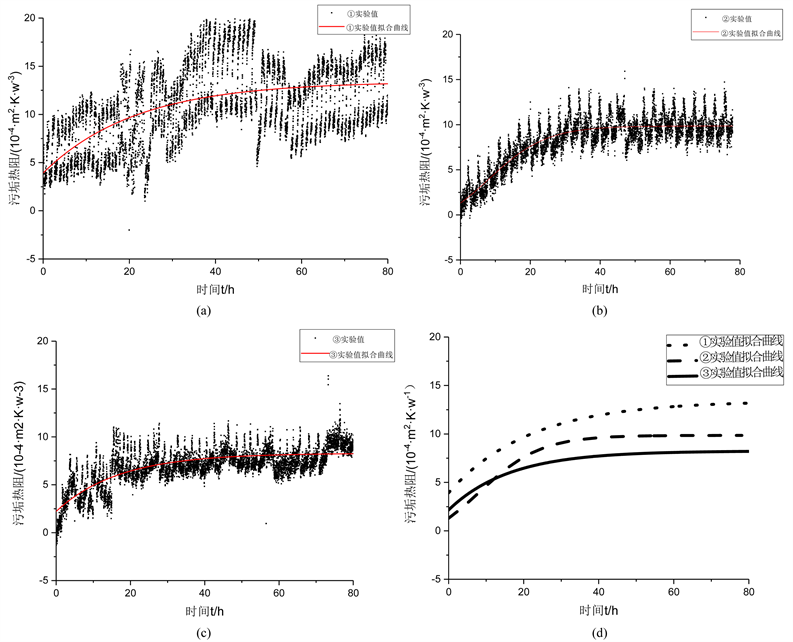Figure 3. (a) The change of fouling resistance with time when the temperature of the thermal cycle is 21˚C; The change of fouling resistance with time when the temperature of the thermal cycle is 26˚C; (c) The change of fouling resistance with time when the temperature of the thermal cycle is 31˚C; (d) Experimental fitting curve of thermal resistance at different thermal cycle inlet temperature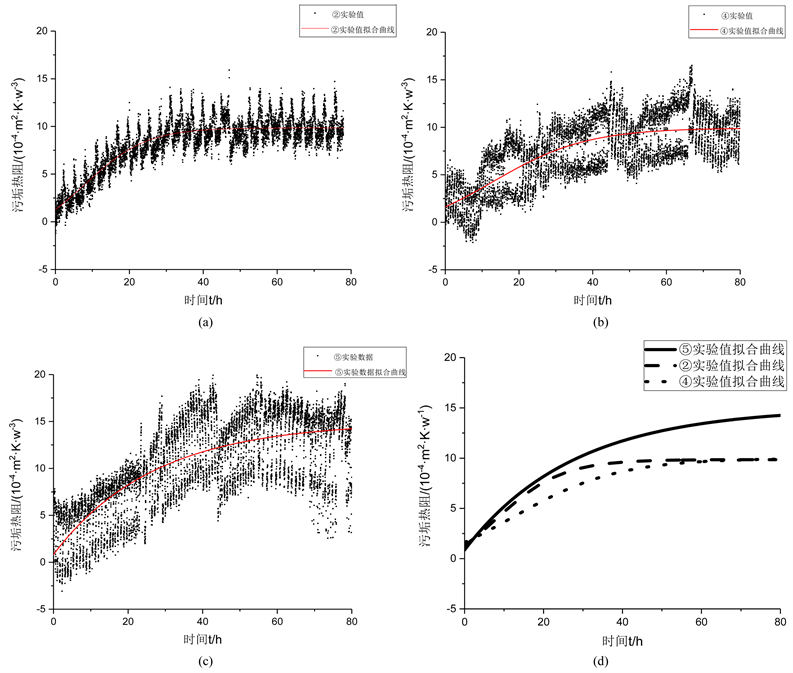Figure 4. (a) The change of fouling thermal resistance with time when the cold cycle inlet temperature is 14˚C; (b) The change of fouling thermal resistance with time when the cold cycle inlet temperature is 16˚C; (c) The change of fouling thermal resistance with time when the cold cycle inlet temperature is 18˚C; (d) Experimental fitting curve of temperature fouling resistance test at different cold cycle inlet temperature

3.3. 温度对于污垢质量的影响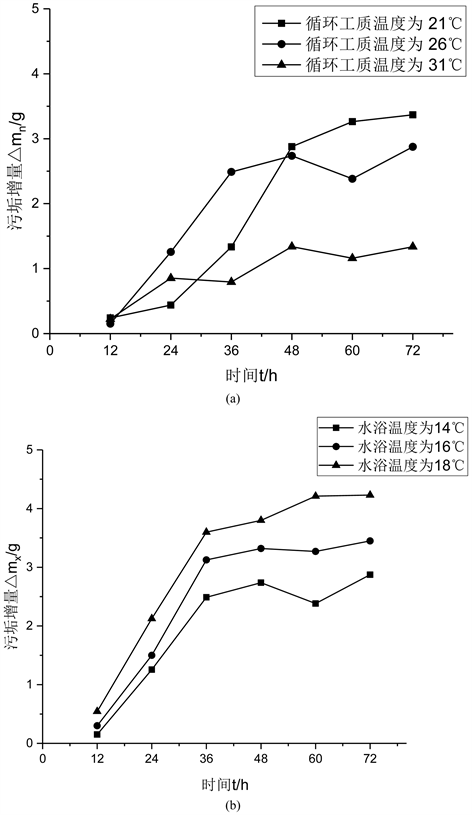Figure 5. (a) Increment line graph of mixed fouling at different time of different thermal cycle; (b) Increment line diagram of mixed fouling at different time in different cold cycle water bath temperature

4. 结论

1) 温度对于以二氧化硅和铁细菌为代表的混合污垢影响明显，并且呈现一定规律，尤其是混合污垢热阻渐近值以及达到渐近值的时间，其中微生物污垢成分在此变化规律中作用明显。虽然热循环温度和冷循环温度对于混合污垢热阻的影响略有不同，但均表现出明显的趋热性。

2) 冷热循环温度对于混合污垢增量影响明显，且与污垢热阻法得出结论大致相同，但是由于微生物含水量高，密度较小等因素，改变冷循环温度对于混合污垢增量影响较小，因此综合考虑混合污垢中各部分的性质对于混合污垢研究有重要作用。

  Hasan, B.O., Nathan, G.J., Ashman, P.J., et al. (2012) The Effects of Temperature and Hydrodynamics on the Crystallization Fouling under Cross Flow Conditions. Applied Thermal Engineering, 36, 210-218. https://doi.org/10.1016/j.applthermaleng.2011.12.027  Al-Otaibi, D.A., Hashmi, M.S.J. and Yilbas, B.S. (2014) Fouling Resistance of Brackish Water: Comparison of Fouling Characteristics of Coated Carbon Steel and Titanium Tubes. Experimental Thermal & Fluid Science, 55, 158-165. https://doi.org/10.1016/j.expthermflusci.2014.03.010  徐志明, 陈洋, 刘坐东, 等. Ca2+环境下板式换热器微生物污垢特性[J]. 化工机械, 2015, 42(5): 615-619.  刘坐东, 陈洋, 王景涛, 等. Ca2+环境下水质参数变化对板式换热器铁细菌微生物污垢的影响[J]. 化工进展, 2016, 35(10): 3344-3349.  陈霄, 杨启容, 张宁, 等. 城市污水换热设备中铁细菌生长特性实验研究[J]. 青岛大学学报(自然科学版), 2016, 29(4): 34-37.  关晓辉, 崔长龙, 曹生现, 等. 不锈钢缩放管中典型致垢微生物致垢能力[J]. 化工进展, 2013, 32(6): 1429-1434.  徐志明, 王景涛, 王宇航, 等. 温度对纳米氧化镁颗粒污垢特性的影响[J]. 热科学与技术, 2016, 15(1): 52-58.  徐志明, 王景涛, 王磊, 等. 交叉缩放椭圆管颗粒污垢特性的实验分析[J]. 化工进展, 2014, 33(4): 831-836.  徐志明, 张仲彬, 孙灵芳, 等. 析晶与颗粒混合污垢的实验研究[J]. 工程热物理学报, 2005, 26(5): 853-855.  车海燕, 徐章法. 敞开式循环冷却水系统中的微生物及其控制[J]. 上海化工, 2005, 30(6): 4-7.